# Excercise 3.4 Understanding Quadrilaterals- NCERT Solutions Class 8

## Chapter 3 Ex.3.4 Question 1

State whether True or False.

(a) All rectangles are squares.

(b) All rhombuses are parallelograms.

(c) All squares are rhombuses and also rectangles.

(d) All squares are not parallelograms.

(e) All kites are rhombuses.

(f) All rhombuses are kites.

(g)All parallelograms are trapeziums.

(h) All squares are trapeziums.

### Solution

Steps:

 Shapes True or False Reason A All rectangles are Squares. False A rectangle need not have all sides equal hence it is not square. B All rhombuses are parallelograms True Since the opposite sides of a rhombus have the same length, it is also a parallelogram C All squares are rhombuses and also rectangles. True All squares are rhombuses as all sides of a square are of equal lengths. A square is a rectangle as each internal angle is $$90$$ degrees. D All squares are not parallelograms. False The opposite sides of a parallelogram are of equal length hence squares with all sides equal are paralleolgrams. E All kites are Rhombuses. False Since rhombus have all sides of equal length. All kites need not have all sides of the same length. F All rhombuses are kites. True Since, all rhombuses have equal sides and diagonals bisect each other. G All parallelograms are trapeziums True Since, trapezium has only two parallel sides. H All squares are Trapeziums. True All trapezium have a pair of parallel sides hence squares can be trapezium.

## Chapter 3 Ex.3.4 Question 2

Identify all the quadrilaterals that have.

(a) four sides of equal length

(b) four right angles

### Solution

Steps:

a) Four sides of equal length - Rhombus and Square are the quadrilaterals with $$4$$ sides of equal length.

b) Four right angles - Square and Rectangle are the quadrilaterals with $$4$$ right angles.

## Chapter 3 Ex.3.4 Question 3

Explain how a square is.

(ii) a parallelogram

(iii) a rhombus

(iv) a rectangle

### Solution

Steps:

 (i) Quadrilateral A square is a quadrilateral since it has four sides. (ii) Parallelogram- properties (1) Opposite sides are equal. (2) Opposite angles are equal. (3) Diagonals bisect one another. A square is parallelogram, since it contains both pairs of opposite sides equal. (iii) Rhombus - properties i)A parallelogram with sides of equal length. ii)The diagonals of a rhombus are perpendicular bisectors of one another. A square is a rhombus since i) its four sides are of same length. ii)the diagonals of a square are perpendicular bisectors of each other. (iv) Rectangle-properties i)Being a parallelogram, the rectangle has opposite sides of equal length and its diagonals bisect each other. A square is rectangle since each interior angle measures $$90$$ degree.

## Chapter 3 Ex.3.4 Question 4

(i) bisect each other

(ii) are perpendicular bisectors of each other

(iii) are equal

### Solution

Steps:

(i) bisect each other

Parallelogram: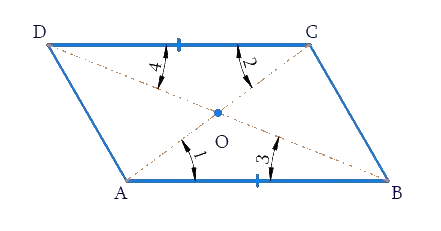Rhombus: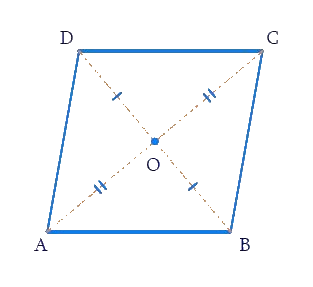Rectangle: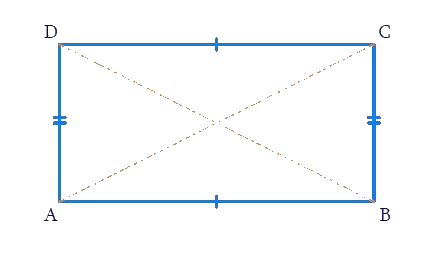Square: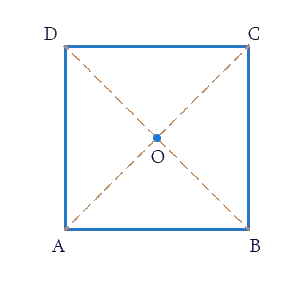a) Parallelogram

b) Rhombus

c) Rectangle

d) Square

The diagonals of a parallelogram, rhombus, rectangle and square are perpendicular bisectors of each other.

ii) Are perpendicular bisectors of each other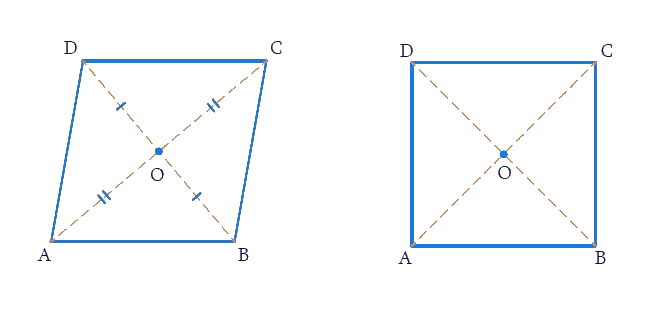a) Rhombus

b) Square

The diagonals of a square and rhombus are perpendicular bisectors of each other.

(iii) are equal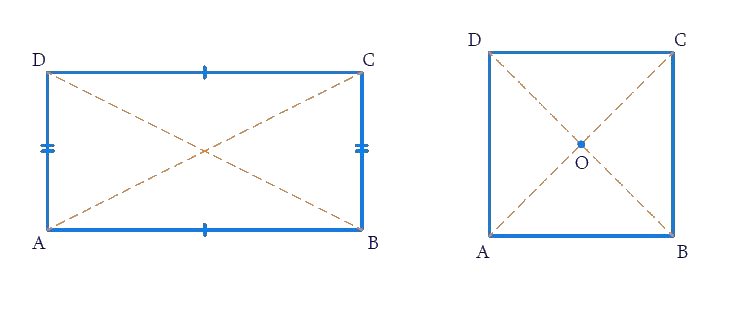a) Rectangle

b) Square

The diagonals of a rectangle and square are equal.

## Chapter 3 Ex.3.4 Question 5

Explain why a rectangle is a convex quadrilateral.

### Solution

Steps:

Polygons that are convex have no portions of their diagonals in their exteriors. A rectangle is a convex quadrilateral since its vertex are raised and both of its diagonals lie in its interior.

Or

None of the angles being a reflex angle, So rectangle is convex quadrilateral.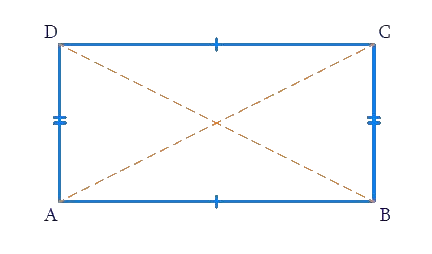## Chapter 3 Ex.3.4 Question 6

$$ABC$$ is a right-angled triangle and $$O$$ is the midpoint of the side opposite to the right angle. Explain why $$O$$ is equidistant from $$A, B$$ and $$C$$. (The dotted lines are drawn additionally to help you).### Solution

What is Known?

$$ABC$$ is a right-angled triangle and $$O$$ is the midpoint of the side opposite to the right angle.

What is Unknown?

Why $$O$$ is equidistant from $$A, B$$ and $$C$$

Reasoning:

Since, two right triangles make a rectangle and in any rectangle, diagonals bisect each other.

Steps:

$$ABCD$$ is a rectangle as opposite sides are equal and parallel to each other and all the interior angles are of $$90^\circ .$$

\begin{align}{\rm{AD}}\left| {\left| {{\rm{BC}},{\rm{AB}}} \right|} \right|{\rm{DC}}\\{\rm{AD }} = {\rm{BC}},{\rm{ AB }} = {\rm{ DC}}\end{align}

In a rectangle, diagonals are of equal length and also these bisect each other.

Hence, $${\rm{AO }} = {\rm{ OC }} = {\rm{ BO }} = {\rm{ OD}}$$

Since, two right triangles make a rectangle where $$O$$ is equidistant point from $$A, B, C$$ and $$D$$ because $$O$$ is the mid-point of the two diagonals of a rectangle.

So, $$O$$ is equidistant from $$A, B, C$$ and $$D.$$

Related Sections
Related Sections
Instant doubt clearing with Cuemath Advanced Math Program